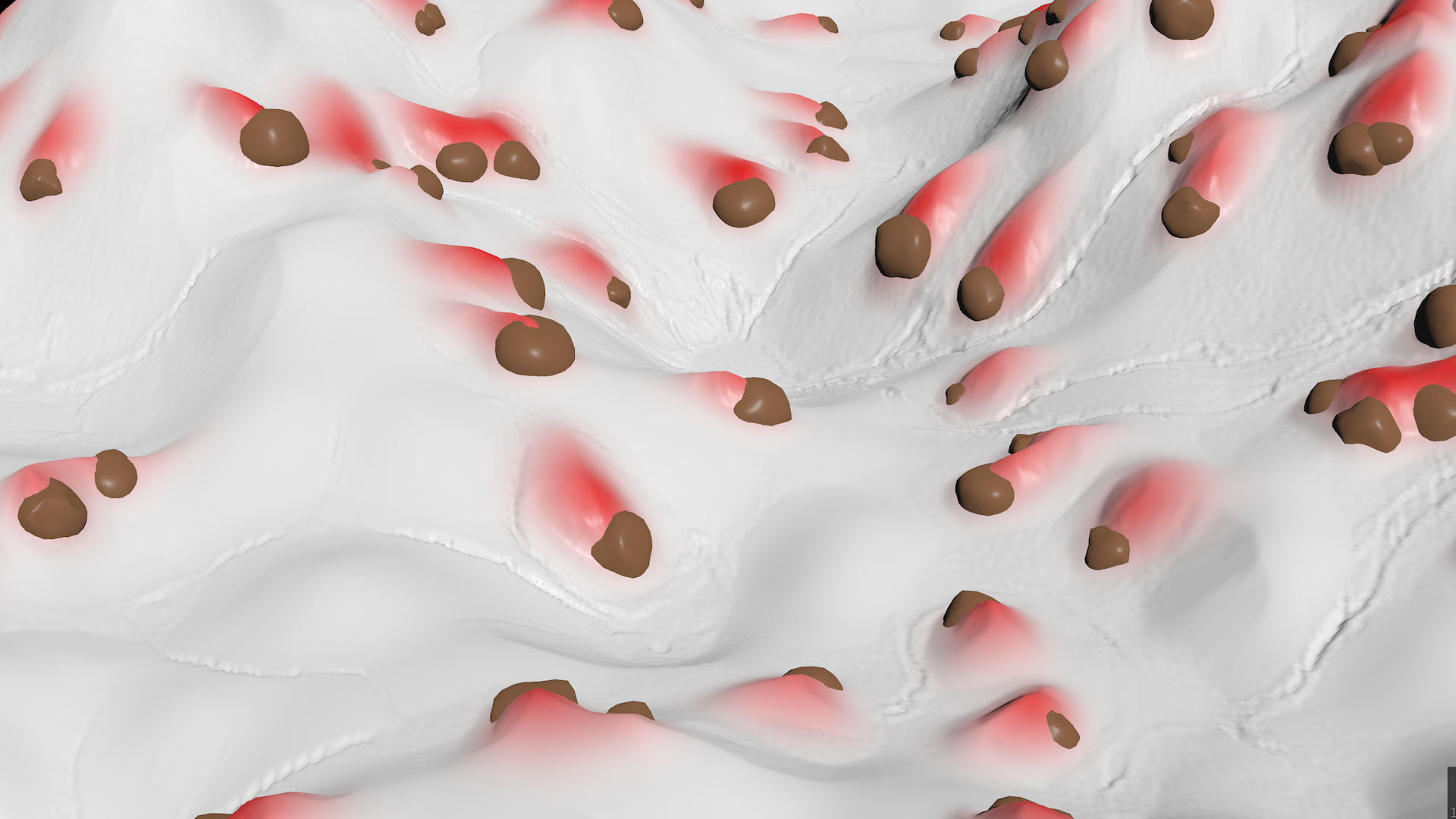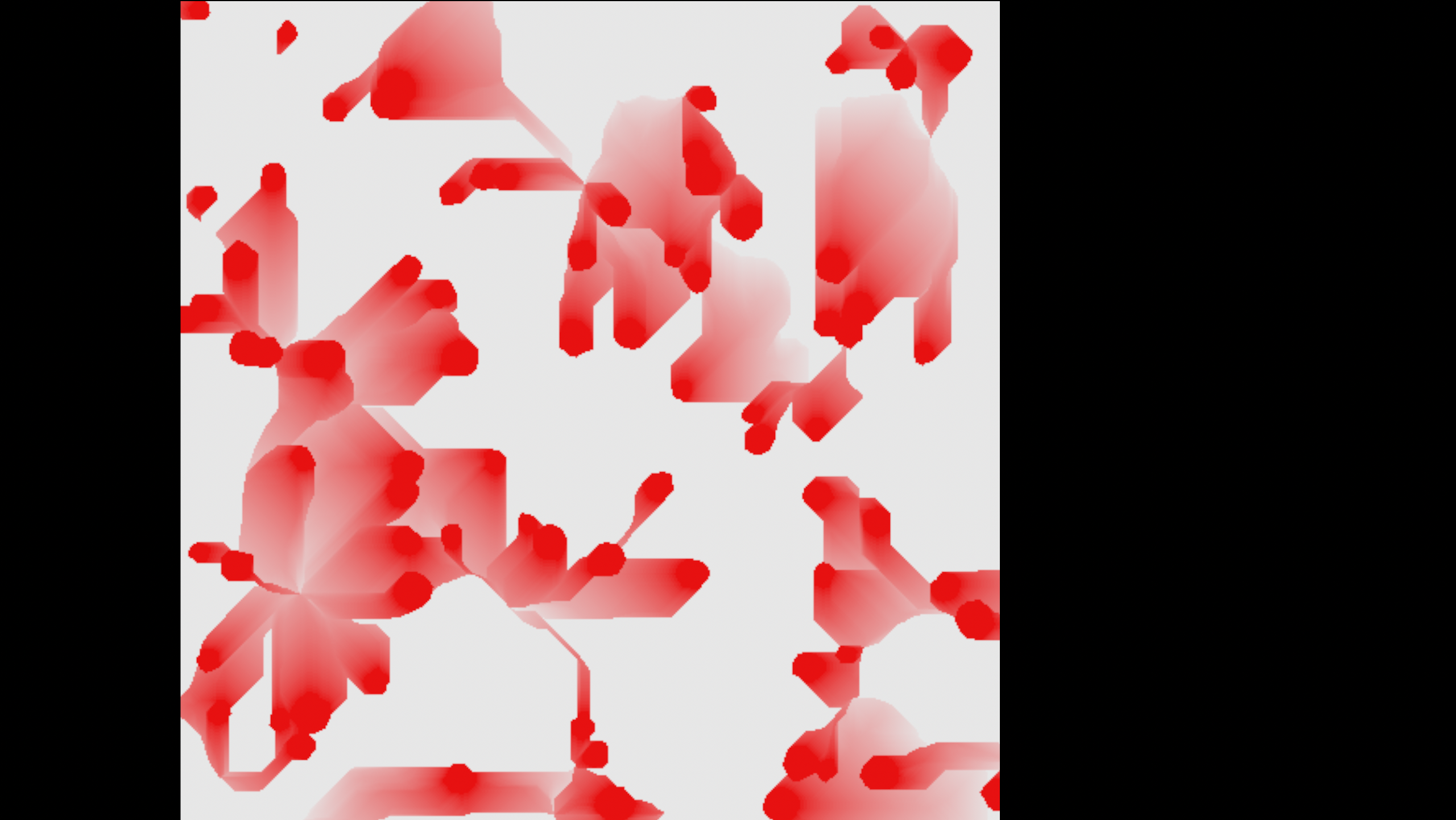# Accumulate snow behind rocks

calendar_today

11.03.2023

label

Environment

mouse

Houdini 19.5

### 1 Description

Streaking the object mask along the reversed gradient to displace the height field areas above the rocks.

### 2 Code discussionFor heigth fields the `volumegradient` is the direction up the hill, thus negating the `normalize(grad)` is used to offset the sampling position downwards. The `volumesample` then checks if the mask is painted at that position with `if(sample > 0.1)`. If it is painted, then the actual position (above the mask) is painted, as well, albeit with fading strength defined by u which is the division of current `iter`ation by the total `num`ber of iterations.

``````vector grad = volumegradient(0, 'height', v@P);
vector pos = v@P - normalize(grad) * 2.5;
float sample = volumesample(0, 'mask', pos);

int iter = detail(1, 'iteration', 0);
int num = detail(1, 'numiterations', 0);
float u = 1.0 - iter / (num - 1.0);

### 3 Alternative

snow_accum.hipnc contains a more elegant approach:

``````int steps = chi('steps');
float w = chf('width');

float dist = 0.0;
vector pos = v@P;
for(int i = 0; i < steps; i++){